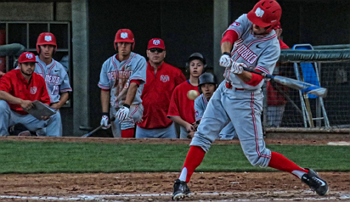# Project 2There are 5 boys who throw the ball at different angles from the ground.

A throws at $30^\circ$.
B throws at $45^\circ$.
C throws at $15^\circ$.
D throws at $90^\circ$.
E throws at $60^\circ$.

Whose ball will cover maximum horizontal distance?

×